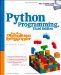# Sorting Sequences

Another staple of many systems is sorting: ordering items in a collection according to some constraint. The script in Example 17-24 defines a simple sort routine in Python, which orders a list of objects on a field. Because Python indexing is generic, the field can be an index or key -- this function can sort lists of either sequences or mappings.

Example 17-24. PP2EDstructClassicssort1.py

```def sort(list, field):
res = [] # always returns a list
for x in list:
i = 0
for y in res:
if x[field] <= y[field]: break # list node goes here?
i = i+1
res[i:i] = [x] # insert in result slot
return res

if __name__ == '__main__':
table = [ {'name':'john', 'age':25}, {'name':'doe', 'age':32} ]
print sort(table, 'name')
print sort(table, 'age')
table = [ ('john', 25), ('doe', 32) ]
print sort(table, 0)
print sort(table, 1)```

Here is this module's self-test code in action:

```C:...PP2EDstructClassics>python sort1.py
[{'age': 32, 'name': 'doe'}, {'age': 25, 'name': 'john'}]
[{'age': 25, 'name': 'john'}, {'age': 32, 'name': 'doe'}]
[('doe', 32), ('john', 25)]
[('john', 25), ('doe', 32)]```

Since functions can be passed in like any other object, we can easily allow for an optional comparison function. In the next version (Example 17-25), the second argument takes a function that should return true if its first argument should be placed before its second. A lambda is used to provide an ascending-order test by default. This sorter also returns a new sequence that is the same type as the sequence passed in, by applying the slicing techniques used in earlier sections: if you sort a tuple of nodes, you get back a tuple.

Example 17-25. PP2EDstructClassicssort2.py

```def sort(seq, func=(lambda x,y: x <= y)): # default: ascending
res = seq[:0] # return seq's type
for j in range(len(seq)):
i = 0
for y in res:
if func(seq[j], y): break
i = i+1
res = res[:i] + seq[j:j+1] + res[i:] # seq can be immutable
return res

if __name__ == '__main__':
table = ({'name':'doe'}, {'name':'john'})
print sort(list(table), (lambda x, y: x['name'] > y['name']))
print sort(tuple(table), (lambda x, y: x['name'] <= y['name']))
print sort('axbyzc')```

This time, the table entries are ordered per a field comparison function passed in:

```C:...PP2EDstructClassics>python sort2.py
[{'name': 'john'}, {'name': 'doe'}]
({'name': 'doe'}, {'name': 'john'})
abcxyz```

This version also dispenses with the notion of a field altogether and lets the passed-in function handle indexing if needed. That makes this version much more general; for instance, it's also useful for sorting strings.Python Programming for the Absolute Beginner, 3rd Edition
ISBN: 1435455002
EAN: 2147483647
Year: 2000
Pages: 245

Similar book on Amazon